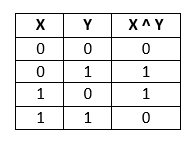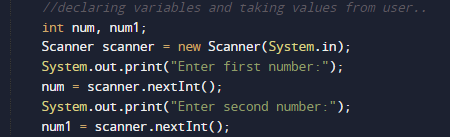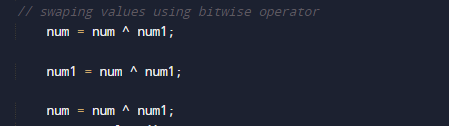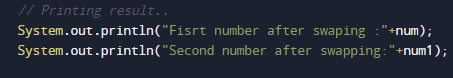# Java Program to swap two numbers using bitwise operator

In this tutorial you will learn about the Java Program to swap two numbers using bitwise operator and its application with practical example.

In this tutorial, we will learn to create a Java Program to swap two numbers using bitwise operator using Java programming.

## Prerequisites

Before starting with this tutorial we assume that you are best aware of the following Java programming topics:

• Java Operators.
• Basic Input and Output function in Java.
• Class and Object in Java.
• Basic Java programming.
• If-else statements in Java.
• For loop in Java.

## What is Bitwise Operator.

Bitwise operator XOR is used to swap two numbers. It’s symbol is  (^). It checks bits of two operands and returns “false” or “0″ if they are equal and returns “true or 1″ if they are not equal. The truth table of  XOR operator is as follows:x = 5, y = 6
x = x ^ y -> x =11
y = x ^ y -> y = 5
x = x ^ y -> x = 6

## Java Program to swap two numbers using bitwise operator

In this program we would swap two values using bitwise Operator .first of we would take a values from user and then swap them using bitwise Operator. Let’s have a look at Code.

## OutputIn the above program, we have first declared and initialized a set variables required in the program.

• no,number= it will hold entered number.
• Sum=   it will hold result of calculation.

After that we take a numbers from user and we will swap value using bitwise operator.• First of all we find binary equivalent of given variables.( num and num1).
• Find num = num ^ num1.
• Again, find  num1 = num ^ num1.
• Find num^num1  and store it in num
• i.e. num = num ^ num1.
• Numbers are swapped now.As shown in image values of number are being swapped using Bitwise operator.and then finally we will print swapped values of numbers.

In this tutorial we have learn about the Java Program to swap two numbers using bitwise operator and its application with practical example. I hope you will like this tutorial.